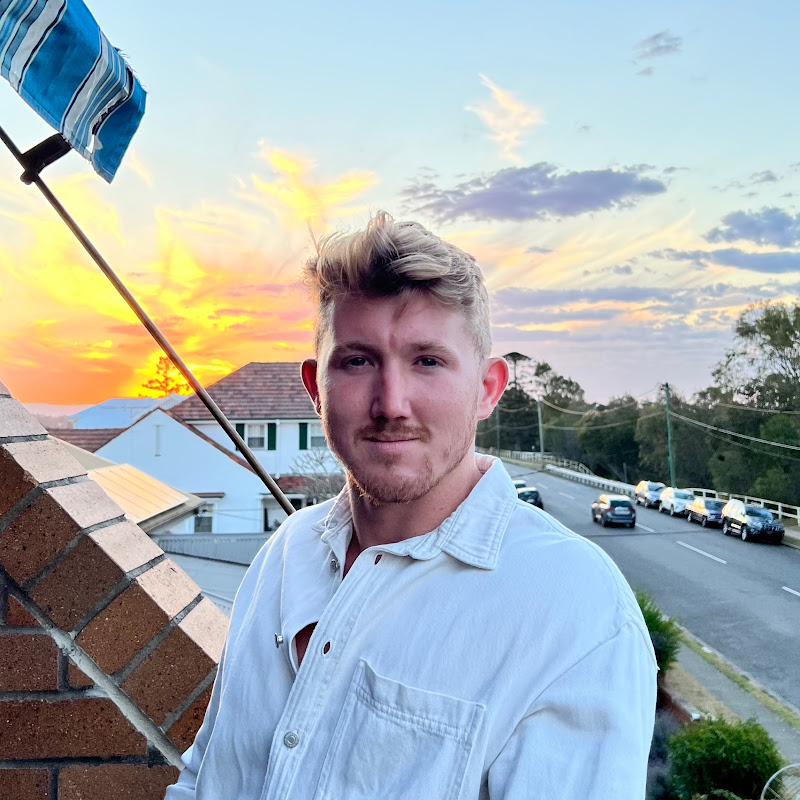# Learn PyTorch for deep learning in a day. Literally.##### Daniel Bourke

Welcome to the most beginner-friendly place on the internet to learn PyTorch for deep learning.

All code on GitHub – https://dbourke.link/pt-github
Read the course materials online – https://learnpytorch.io

Below are the timestamps/outline of the video. The video you’re watching is comprised of 162 smaller videos but YouTube limits timestamps at 100 so some have been left out.

00:00 Hello 🙂

🛠 Chapter 0: PyTorch Fundamentals
01:17 0. Welcome and “what is deep learning?”
07:13 1. Why use machine/deep learning?
10:47 2. The number one rule of ML
16:27 3. Machine learning vs deep learning
22:34 4. Anatomy of neural networks
36:28 6. What can deep learning be used for?
42:50 7. What is/why PyTorch?
53:05 8. What are tensors?
57:24 9. Outline
1:03:28 10. How to (and how not to) approach this course
1:08:37 11. Important resources
1:14:00 12. Getting setup
1:21:40 13. Introduction to tensors
1:35:07 14. Creating tensors
1:53:33 17. Tensor datatypes
2:02:58 18. Tensor attributes (information about tensors)
2:11:22 19. Manipulating tensors
2:17:22 20. Matrix multiplication
2:47:50 23. Finding the min, max, mean and sum
2:57:20 25. Reshaping, viewing and stacking
3:11:03 26. Squeezing, unsqueezing and permuting
3:23:00 27. Selecting data (indexing)
3:32:33 28. PyTorch and NumPy
3:41:42 29. Reproducibility
3:52:30 30. Accessing a GPU
4:04:21 31. Setting up device agnostic code

🗺 Chapter 1: PyTorch Workflow
4:16:59 33. Introduction to PyTorch Workflow
4:19:46 34. Getting setup
4:27:02 35. Creating a dataset with linear regression
4:36:44 36. Creating training and test sets (the most important concept in ML)
4:52:50 38. Creating our first PyTorch model
5:13:13 40. Discussing important model building classes
5:19:41 41. Checking out the internals of our model
5:29:33 42. Making predictions with our model
5:40:47 43. Training a model with PyTorch (intuition building)
5:49:03 44. Setting up a loss function and optimizer
6:01:56 45. PyTorch training loop intuition
6:39:37 48. Running our training loop epoch by epoch
6:49:03 49. Writing testing loop code
7:44:00 54. Putting everything together

🤨 Chapter 2: Neural Network Classification
8:31:32 60. Introduction to machine learning classification
8:41:14 61. Classification input and outputs
8:50:22 62. Architecture of a classification neural network
9:09:13 64. Turing our data into tensors
9:25:30 66. Coding a neural network for classification data
9:43:27 68. Using torch.nn.Sequential
9:56:45 69. Loss, optimizer and evaluation functions for classification
10:11:37 70. From model logits to prediction probabilities to prediction labels
10:27:45 71. Train and test loops
10:57:27 73. Discussing options to improve a model
11:27:24 76. Creating a straight line dataset
11:45:34 78. Evaluating our model’s predictions
11:50:58 79. The missing piece: non-linearity
12:42:04 84. Putting it all together with a multiclass problem
13:23:41 88. Troubleshooting a mutli-class model

😎 Chapter 3: Computer Vision
14:00:20 92. Introduction to computer vision
14:12:08 93. Computer vision input and outputs
14:22:18 94. What is a convolutional neural network?
14:27:21 95. TorchVision
14:36:42 96. Getting a computer vision dataset
15:01:06 98. Mini-batches
15:51:33 103. Training and testing loops for batched data
16:25:59 105. Running experiments on the GPU
16:29:46 106. Creating a model with non-linear functions
16:41:55 108. Creating a train/test loop
17:13:04 112. Convolutional neural networks (overview)
17:21:29 113. Coding a CNN
17:41:18 114. Breaking down nn.Conv2d/nn.MaxPool2d
18:28:34 118. Training our first CNN
18:43:54 120. Making predictions on random test samples
18:55:33 121. Plotting our best model predictions
19:19:06 123. Evaluating model predictions with a confusion matrix

🗃 Chapter 4: Custom Datasets
19:43:37 126. Introduction to custom datasets
20:13:31 129. Becoming one with the data
20:38:43 132. Turning images into tensors
21:24:52 137. Creating a custom dataset class (overview)
21:42:01 139. Writing a custom dataset class from scratch
22:21:22 142. Turning custom datasets into DataLoaders
22:28:22 143. Data augmentation
22:42:46 144. Building a baseline model
23:10:39 147. Getting a summary of our model with torchinfo
23:17:18 148. Creating training and testing loop functions
23:50:31 151. Plotting model 0 loss curves
23:59:34 152. Overfitting and underfitting
24:32:03 155. Plotting model 1 loss curves
24:35:25 156. Plotting all the loss curves
24:46:22 157. Predicting on custom data

#pytorch #machinelearning #deeplearning